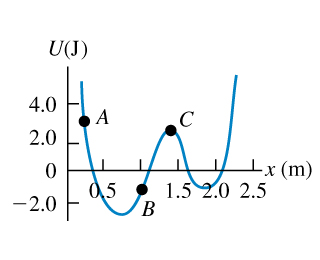# Problem: A particle moves along the x-axis while acted on by a single conservative force parallel to the x-axis. The force corresponds to the potential-energy function graphed in the figure . The particle is released from rest at point exttip{A}{A}.What is the direction of the force on the particle when it is at point exttip{A}{A}?At point exttip{B}{B}?At what value of exttip{x}{x} is the kinetic energy of the particle a maximum?What is the force on the particle when it is at point C?What is the largest value of exttip{x}{x} reached by the particle during its motion?What value or values of exttip{x}{x} correspond to points of stable equilibrium?Of unstable equilibrium?

###### FREE Expert Solution
89% (141 ratings)
###### Problem Details

A particle moves along the x-axis while acted on by a single conservative force parallel to the x-axis. The force corresponds to the potential-energy function graphed in the figure. The particle is released from rest at point .

What is the direction of the force on the particle when it is at point ?

At point ?

At what value of is the kinetic energy of the particle a maximum?

What is the force on the particle when it is at point C?

What is the largest value of reached by the particle during its motion?

What value or values of correspond to points of stable equilibrium?

Of unstable equilibrium?